Latest Banking jobs   »

# Quantitative Aptitude Quiz For LIC AAO Mains 2023- 11th March

Directions (1-5): Read the given information carefully and answer the following questions.

Arun & Veer invested Rs. 60,000 and Rs. 90,000 respectively. Arun invested for 15 months while Veer invested for 8 months and the difference between profit share of Arun and Veer is Rs.10000. Arun invested his profit share at 20% p.a on CI for 1.5 years and he bought a bike from the amount he received. Veer bought two laptops L₁ & L₂ (both laptops have equal price) from the amount which he received after investing his profit share at 15% p.a on SI for 2 years. Veer sold L₁ & L₂ at 10% and 15% profit respectively while Arun sold his bike at 5% loss.

Q1. Veer invested money (which he gets from the profit of that two laptops) in a scheme at the rate of 10% p.a on simple interest for 4 years. What is the interest he received?
(a) Rs. 2800
(b) Rs. 2600
(c) Rs. 2500
(d) Rs. 2750
(e) Rs. 3000
Q2. If the price of Bike depreciates 15% every year, then what will be the price of bike after 2 years?
(a) Rs. 47,685
(b) Rs. 49,675
(c) Rs. 48,025
(d) Rs. 47,515
(e) Rs. 48,195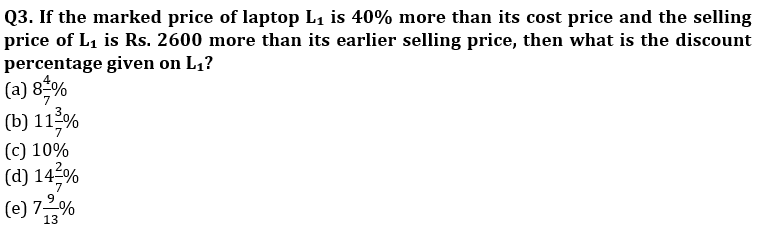Q4. The total amount received by Arun on selling that bike and the total amount received by veer on selling both the laptops together is how much more or less than the 108% of total cost price of all the three items together?
(a) Rs. 6040
(b) Rs. 6180
(c) Rs. 6240
(d) Rs. 6340
(e) Rs. 6380
Q5. In the above passage, if the difference between profit share of Arun and Veer is Rs. 11000 instead of Rs. 10000, then what will be the change in the net profit earned on all the three items together? (Rest all information remains same)
(a) Rs. 120
(b) Rs. 320
(c) Rs. 220
(d) Rs. 520
(e) Rs. 420

Directions (6-10): What will come in place of (?) in the following questions?

Q6. 81, ?, 130, 147, 179, 194
(a) 108
(b) 100
(c) 115
(d) 111
(e) 104

Q7. ?, 378, 174, 438, 106, 514
(a) 154
(b) 172
(c) 128
(d) 226
(e) 202

Q8. 16, ?, 80, 172, 360, 740
(a) 48
(b) 52
(c) 36
(d) 44
(e) 40

Q9. 44, 106, 180, 262, ?, 442
(a) 474
(b) 498
(c) 466
(d) 484
(e)348

Q10. 64, 142, ?, 532, 844, 1234
(a) 312
(b) 364
(c) 488
(d) 436
(e) 298
Q11. ‘X’ is a perfect square less than 40, and ‘Y’ is a perfect cube less than 100.
Quantity I – What will be probability of X > Y.
Quantity II – What will be probability that the sum of X & Y is an odd number.
(a) Quantity I > Quantity II
(b) Quantity I ≥ Quantity II
(c) Quantity II > Quantity I
(d) Quantity II ≥ Quantity I
(e) Quantity I = Quantity II or Relation cannot be established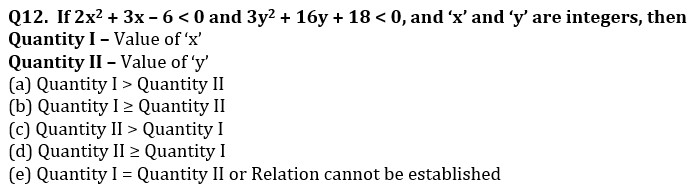Q13. Speed of boat Q in still water is 25% less than that of boat P and speed of current is 4 km/hr. Boat Q starts from point A in downstream, two hours earlier than boat P and it is found that boat P is 16 km ahead of boat Q after ‘t’ hours of its start. If maximum speed of boat P in still water can be 16 km/hr, then for which of following value of ‘t’, speed of boat Q in upstream will have integral value?
(i) 10 h
(ii) 9 h
(iii) 12 h
(iv) 14 h
(v) 15 h
(a) Only I & III
(b) Only II, IV & V
(c) Only III, IV, & V
(d) Only III & IV
(e) Only IV & V

Directions (14-15): Time taken by car P to cover a certain distance is 50% more than the time taken by car Q to cover the same distance. Car P and another car R starts simultaneously from a same point in same direction. After 1 hour, car Q starts in the same direction as that of car P and R. Q overtakes P in ‘x’ hours and R in ‘x+1.5’ hours.

Q14. If speed of car P is 120 km/hr, then what is the speed of car R.
(a) 120 km/hr
(b) 140 km/hr
(c) 100 km/hr
(d) 75 km/hr
(e) 80 km/hr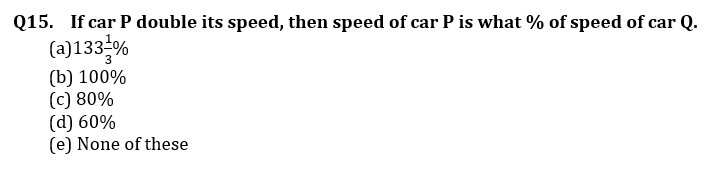Solutions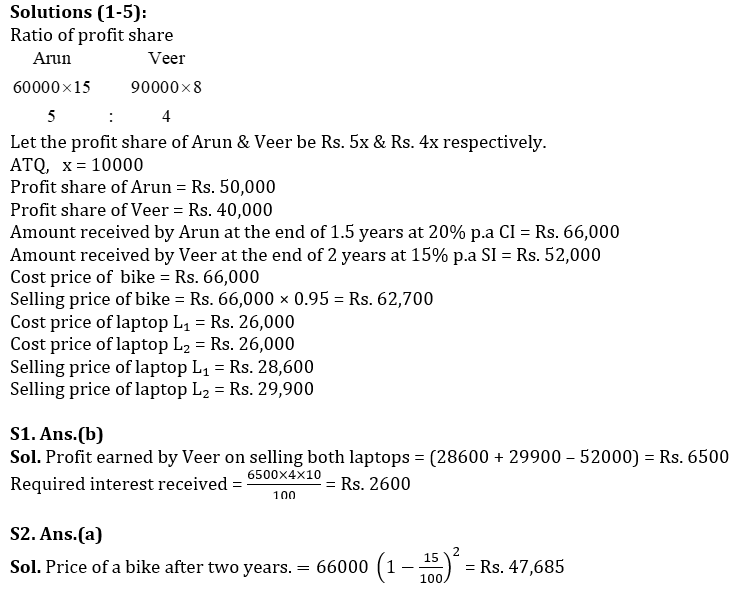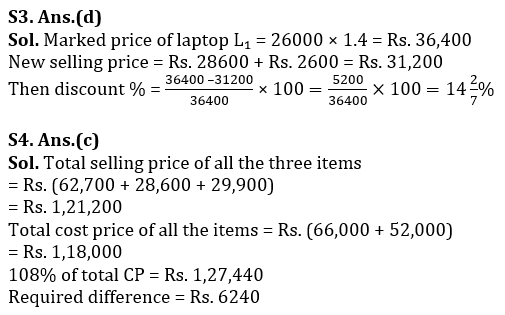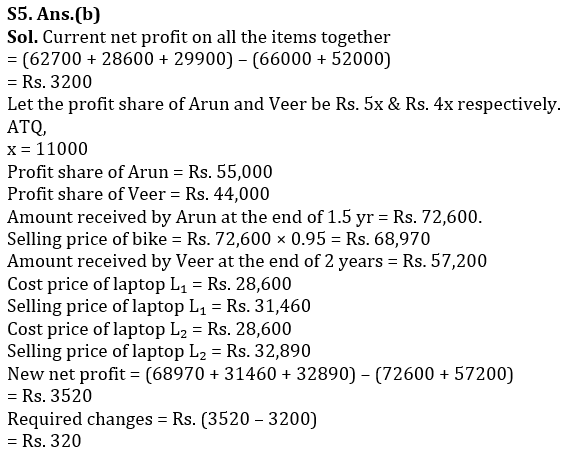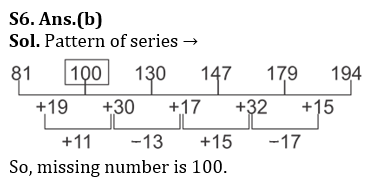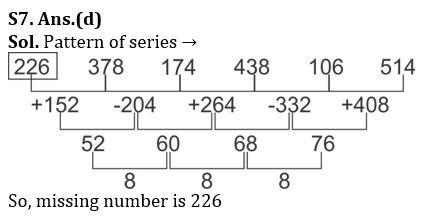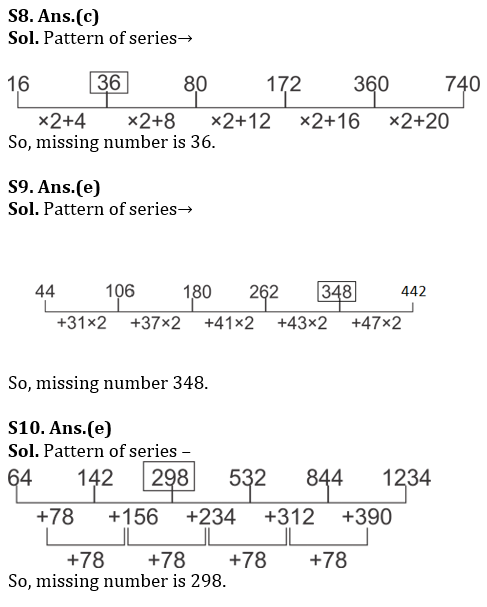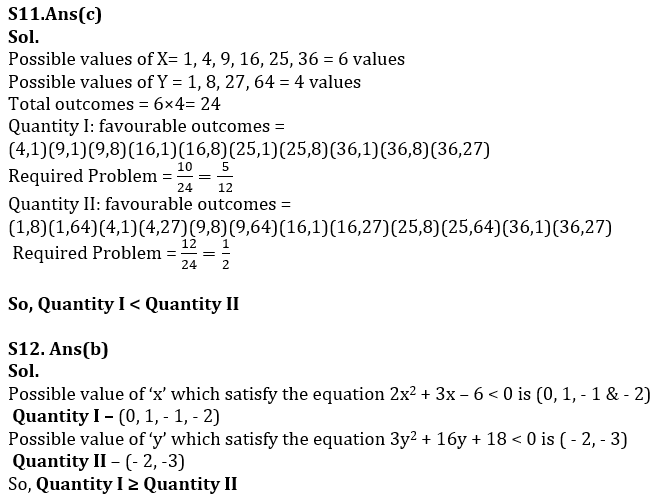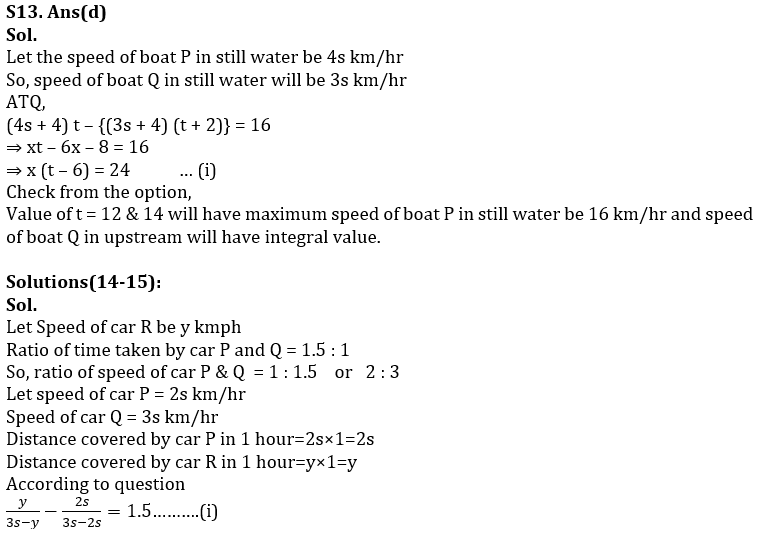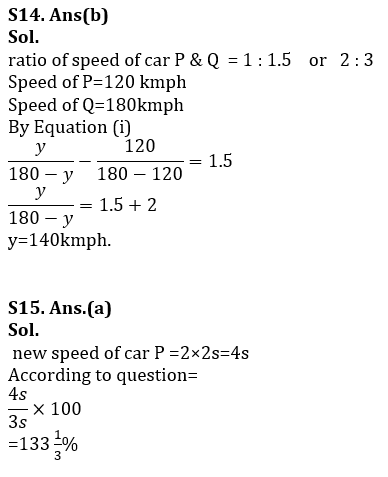## FAQs

### When will the LIC AAO Mains exam be held?

LIC AAO Mains exam will be held on 18 March

#### Congratulations!Union Budget 2023-24: Free PDF